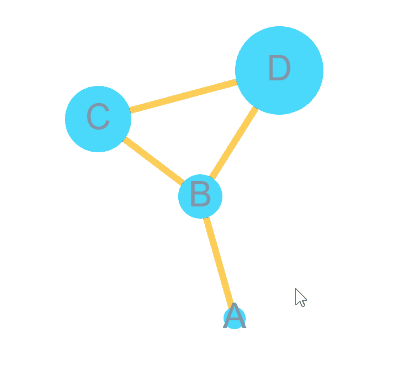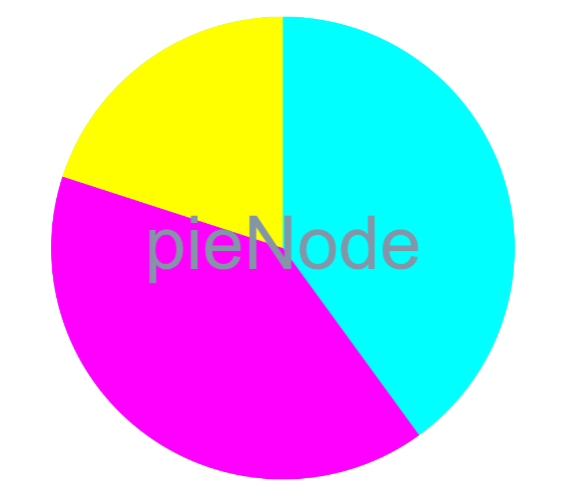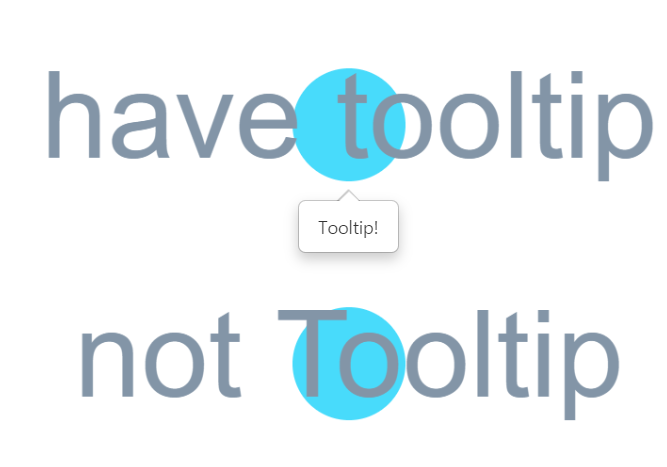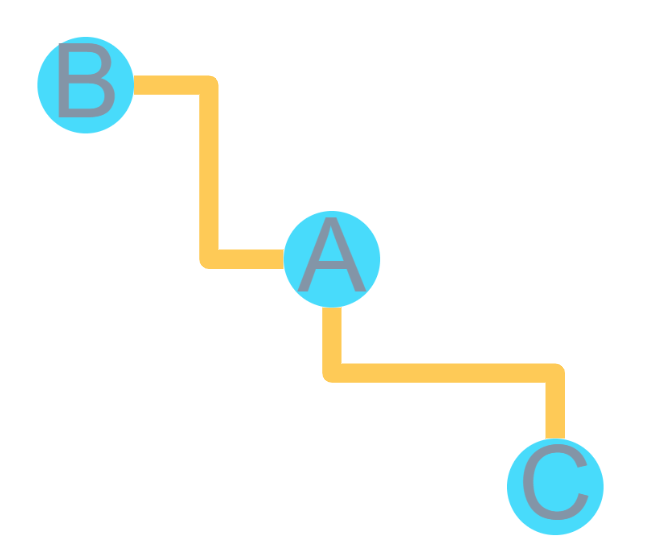# unistbig/shinyCyJS

No description, website, or topics provided.
JavaScript RFetching latest commit…
Cannot retrieve the latest commit at this time.
Type Name Latest commit message Commit time
Failed to load latest commit information.PNGRSVGinst/htmlwidgets.Rbuildignore.gitattributes.gitignoreDESCRIPTIONLICENSENAMESPACEREADME.mdshinyCyJS.Rproj

# shinyCyJSshinyCyJS is R/Shiny Package to use cytoscape.js in R environment.

cytoscape.js is a great javascript library for visualize/analysis Graph theory ( network )

shinyCyJS is built with cytoscape.js version 3.12.0. (2019/11)

# How to install :

```library(devtools)
install_github('unistbig/shinyCyJS')
library(shinyCyJS)```

# Example :

bulid Graph with node 4 = A, B, C, D and edge = A-B, B-C, C-D, B-D

Code
```library(shiny)
library(shinyCyJS)

ui = function(){
fluidPage(
ShinyCyJSOutput(outputId = 'cy')
)
}

server = function(input, output, session){

nodes = data.frame(
id = c('A','B','C','D'),
width = c(10,20,30,40),
height = c(10,20,30,40)
)

edges = data.frame(
source = c('A','B','C','D'),
target = c('B','C','D','B')
)

nodes = buildElems(nodes, type = 'Node')
edges = buildElems(edges, type = 'Edge')

obj = shinyCyJS(c(nodes, edges))
output\$cy = renderShinyCyJS(obj)
}

shinyApp(ui,server, options = list(launch.browser = TRUE, display.mode ='normal'))
```# Used in

netGO https://github.com/unistbig/netGO
GScluster https://github.com/unistbig/GScluster ( on progress )

# suggest or comment

hwanistic@gmail.com Thanks !

# update log

0.0.2

added pie background feature ( only size and color )demo code :

```shinyCyJS(
buildNode(id = 'pieNode', width = 100, height = 100,
pieSize = c(40,40,20,0,0,0,0,0,0,0,0,0,0,0,0,0),
pieColor = c('cyan','magenta','yellow',rep('#000',13))
)
)```

0.0.3

bugFixed (pre-built elements didn't clear when using rendershinyCyJS)

0.0.4

tooltip feature available on Nodes

for information,

Cytoscape-Qtip https://github.com/cytoscape/cytoscape.js-qtipdemo code :

`shinyCyJS(list( buildNode('have tooltip',tooltip = 'Tooltip!'), buildNode('not Tooltip')) )`

0.0.5

0.0.6 fcose, spread, dagre layout available.
0.0.7

now multiple elements with data.frame can be built with buildElems

below codes will work same

```shinyCyJS(list(
buildNode('a'),
buildNode('b', width = 20),
buildNode('c', width = 30),
buildNode('d', width = 40),
buildEdge('a','b'),
buildEdge('a','c'),
buildEdge('c','d'),
buildEdge('b','d')
))```
```a = data.frame(
id = c('a','b','c','d'),
width = c(15,20,30,40)
)

b = data.frame(
source = c('a','a','c','b'),
target = c('b','c','d','d')
)

nodes = buildElems(a,'Node')
edges = buildElems(b,'Edge')

shinyCyJS(c(nodes, edges))
```

0.0.8 core file ( cytoscape.js ) has updated with recent version. now available with Taxi edgebelow code used to build this figure

```shinyCyJS(list(
buildNode("A"),
buildNode("B"),
buildNode("C"),
buildEdge("A", "B", curveStyle = "taxi"),
buildEdge("A", "C", curveStyle = "taxi")))```